# Various kinds of pseudoprimes

Every condition that all primes satisfy has a corresponding primality test and corresponding class of pseudoprimes.

The most famous example is Fermat’s little theorem. That theorem says that for all primes p, a certain congruence holds. The corresponding notion of pseudoprime is a composite number that also satisfies Fermat’s congruence.

To make a useful primality test, a criterion should be efficient to compute, and the set of pseudoprimes should be small.

## Fermat pseudoprimes

A Fermat pseudoprime base b is a composite number n such that

bn-1 = 1 (mod n).

The smallest example is 341 because

2340 = 1 mod 341

and 341 = 11*31 is composite. More on Fermat pseudoprimes here.

## Wilson pseudoprimes

Fermat’s little theorem leads to a practical primality test, but not all theorems about primes lead to such a practical test. For example, Wilson’s theorem says that if p is prime, then

(p – 1)! = -1 mod p.

A Wilson pseudoprime would be a composite number n such that

(n – 1)! = -1 mod n.

The good news is that the set of Wilson pseudoprimes is small. In fact, it’s empty!

The full statement of Wilson’s theorem is that

(p – 1)! = -1 mod p

if and only if p is prime and so there are no Wilson pseudoprimes.

The bad news is that computing (p – 1)! is more work than testing whether p is prime.

## Euler pseudoprimes

Let p be an odd prime and let b be a positive integer less than p. Then a theorem of Euler says thatwhere the symbol on the right is the Legendre symbol. It looks like a fraction in paretheses, but that’s not what it means. The symbol is defined to be 1 if b is a square mod p and -1 otherwise. It’s an unfortunate but well-established bit of notation.

We could turn Euler’s theorem around and convert it into a primality test by saying that if a number p does not the congruence above, it cannot be prime.

There’s one complication with this: the Legendre symbol is only defined if p is prime. However, there is a generalization of the Legendre symbol, the Jacobi symbol, which is defined as long as the top argument is relatively prime to the bottom argument. Euler pseudoprimes are also known as Euler-Jacobi pseudoprimes.

The Jacobi symbol uses the same notation as the Legendre symbol, but there is no ambiguity because if the bottom argument is prime, the Jacobi symbol equal the Legendre symbol. More on Legendre and Jacobi symbols here.

An odd composite number n is a Euler pseudoprime base b if n and b are relatively prime andwhere the symbol on the right is now the Jacobi symbol.

There are efficient algorithms for computing Jacobi symbols, and so the criterion above is a practical way to prove a number is not prime. But there are Euler pseudoprimes that slip past this test. A small example is n = 9 and b = 10. Euler pseudoprimes are less common than Fermat pseudoprimes.

## Other examples

There are many other examples: Lucas pseudoprimes, elliptic pseudoprimes, etc. Any theorem about prime numbers can be recast as a primality test, and the composite numbers that slip through labeled pseduoprimes relative to that test. The question is whether such a test is useful, i.e. efficient to compute and without too many false positives.

# Finding large pseudoprimes

Fermat’s little theorem says that if p is a prime number, then for any integer b,

bp-1 = 1 (mod p).

This gives a necessary but not sufficient test for a number to be prime. A number that satisfies the equation above but is not prime is called a pseudoprime  for base b. For example, 341 is a pseudoprime base 2 because

2340 = 1 mod 341

and clearly 341 = 11*31.

How might you go about hunting for pseudoprimes? This page gives a way to find pseduoprimes base 2, quoting the following theorem.

If both numbers q and 2q – 1 are primes and n = q(2q-1) then 2n-1 = 1 (mod n) if and only if q is of the form 12k + 1.

Here’s Python code that will find pseudoprimes of a given size in bits.

    from secrets import randbits
from sympy import isprime

def find_pseudoprime(numbits):
n = numbits // 2
while True:
q = randbits(n)
if isprime(q) and q%12 == 1 and isprime(2*q-1):
return q*(2*q-1)

print( find_pseudoprime(256) )


This returned

47362882341088596725068562696893704769436677460225591859092704246296157080253

in under a second.

Clearly the return value of find_pseudoprime is composite since it is computed as the product of two integers. You could confirm it returns a pseudoprime by computing

    pow(2, p-1, p)


on the return value to verify that it equals 1.

***

 Sometimes such a number is called a Fermat pseudoprime to distinguish it from other kinds of pseudo primes, i.e. numbers that satisfy other necessary conditions for being prime without actually being prime. For example, Perrin pseudoprimes. See the next post for more examples.

# Estimating the proportion of smooth numbersA number is said to be “smooth” if all its prime factors are small. To make this precise, a number is said to be y-smooth if it only has prime factors less than or equal to y. So, for example, 1000 is 5-smooth.

The de Bruijn function φ(x, y) counts the number of y-smooth positive integers up to x, and so φ(x, y)/x is the probability that a number between 1 and x is y-smooth.

It turns out that

φ(x, x1/u)/x ≈ 1/uu.

This means, for example, that the proportion of numbers with less than 100 digits whose factors all have less than 20 digits is roughly 1/55 = 1/3125.

Source: The Joy of Factoring

Here’s a little Python code to experiment with this approximation. We’ll look at the proportion of 96-bit numbers whose largest prime factor has at most 32 bits.

    from secrets import randbits
from sympy.ntheory.factor_ import smoothness

smooth = 0
for _ in range(100):
x = randbits(96)
s = smoothness(x)
if s < 2**32:
smooth += 1
print("Num small:", smooth)


The SymPy function smoothness returns a pair, and the first element of the pair is the largest prime dividing its argument.

In our case u = 3, and so we’d expect about 1/27 of our numbers to have no factors larger than 32 bits. I ran this five times, and got 8, 2, 5, 3, and 7. From the approximation above we’d expect results around 4, so our results are not surprising.

# New RSA factoring challenge solved

How hard is it to factor large numbers? And how secure are encryption methods based on the difficulty of factoring large numbers?

The RSA factoring challenges were set up to address these questions. Last year RSA-230 was factored, and this week RSA-240 was factored. This is a 240 digit (795 bit) number, the product of two primes.

Researchers solved two related problems at the same time, factoring RSA-240 and solving a discrete logarithm problem. Together these problems took about 4,000 core-years to solve. It’s not clear from the announcement how long it would have taken just to factor RSA-240 alone.

If you were to rent the computing power used, I imagine the cost would be somewhere in the six figures.

This makes 2048-bit and 3072-bit RSA keys look very conservative. However, the weakest link in RSA encryption is implementation flaws, not the ability to factor big numbers.

Assume for a moment that breaking RSA encryption requires factoring keys. (This may not be true in theory [*] or in practice.) How long would it take to factor a 2048 or 3072 bit key?

The time required to factor a number n using the number field sieve is proportional toHere o(1) roughly means terms that go away as n gets larger. (More on the notation here.) For simplicity we’ll assume we can ignore these terms.

This suggests that factoring a 2048-bit key is 12 orders of magnitude harder than factoring RSA-240, and that factoring a 3072-bit key is 18 orders of magnitude harder.

However, I don’t think anyone believes that breaking RSA with 2048-bit keys would require a quadrillion core-years. If the NSA believed this, they wouldn’t be recommending that everyone move to 3072-bit keys.

Why such a large discrepancy? Here are a few reasons. As mentioned above, RSA encryption often has exploitable implementation flaws. And even if implemented perfectly, there is no proof that breaking RSA encryption is as hard as factoring. And there could be breakthroughs in factoring algorithms. And large-scale quantum computers may become practical, in which case factoring would become much easier.

***

[*] Factoring is sufficient to break RSA, but there’s no proof that it’s necessary. Michael Rabin’s variation on RSA is provably as hard to break as factoring: decryption would enable you to factor the key. But as far as I know, Rabin’s method isn’t used anywhere. Even if you know your method is as hard as factoring, maybe factoring isn’t as hard as it seems. Lower bounds on computational difficulty are much harder to obtain than upper bounds.

# Near zeros of zeta

Alex Kontorovich had an interesting tweet about the Riemann hypothesis a few days ago.

The critical strip of the Riemann zeta function is the portion of the complex plane with real part between 0 and 1. The Riemann hypothesis conjectures that the zeros of the zeta function in this region all have real part 1/2. To put it another way, the Riemann hypothesis says that ζ(x + it) is never zero unless x = 1/2. However, according to Alex Kontorovich’s tweet, the function gets arbitrarily close to zero infinitely often for other values of x.

I wrote a little Mathematica code to produce graphs like the ones above. Here is a plot of ζ(1/2 + it).

    ParametricPlot[
{Re[Zeta[1/2 + I t]], Im[Zeta[1/2 + I t]]},
{t, 0, 100},
AspectRatio -> 1,
PlotRange -> {{-2, 6}, {-3, 3}}
]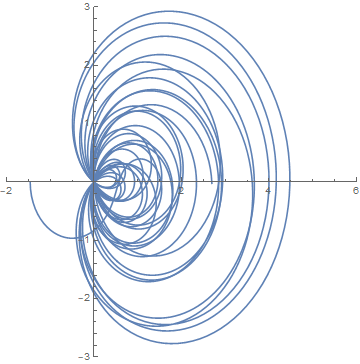At first I didn’t specify the aspect ratio and I got nearly circular arcs. Then I realized that was an artifact of Mathematica’s default aspect ratio. Also, I set the plot range so the image above would be comparable with the next image.

Notice all the lines crossing the origin. These correspond to the zeros of ζ along the line Re(x) = 1/2.

Next I changed the 1/2 to 1/3.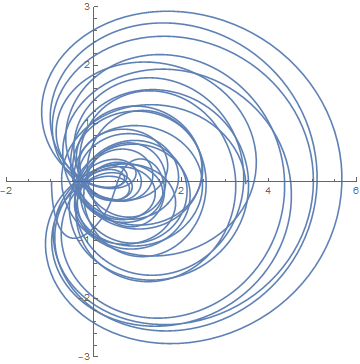If you look closely you’ll see a gap around the origin.

Here’s a closeup of the origin in both graphs. First for x = 1/2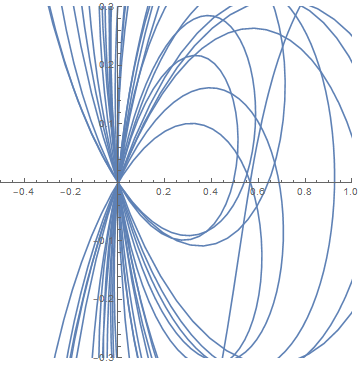and now for x = 1/3.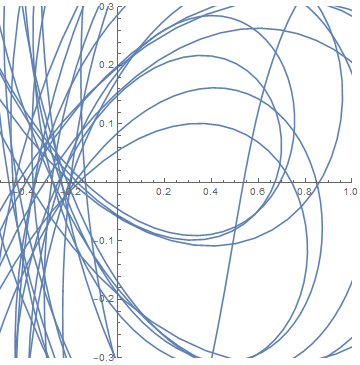I hadn’t seen the result in Kontorovich’s tweet before. I don’t know whether it holds for all x or just for certain values of x. I assume the former. But apparently it at least holds for x = 4/5. So we should be able to find values of |&zeta(4/5 + it)| as small as we like by taking t large enough, and we should always be able to find more such values by looking further out. In practice, you might have to very far out before you find a small value. Looking as far as 1000, for example, it seems the minimum is about 0.2.

    Plot[
Abs[Zeta[4/5 + I t]],
{t, 0, 1000},
PlotRange -> {0, 1}
]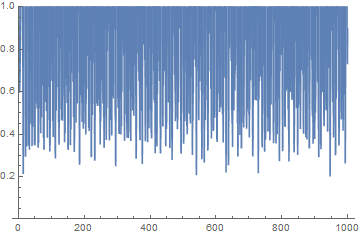If you ask Mathematica to find the minimum in the graph above, it won’t give a reasonable answer; there are too many local minima. If instead you ask it to look repeatedly over small intervals, it does much better. The following minimizes |ζ(4/5 + it)| over the interval [i, i+1].

    f[i_] := FindMinimum[
{Abs[Zeta[4/5 + I t]], i <= t <= i + 1},
{t, i}
]


It returns a pair: the minimum and where it occurred. The following will look for places where |ζ(4/5 + it)| is less than 0.2.

    For[i = 0, i < 1000, i++,
If[ First[f[i]] < 0.20,
Print[f[i]]
]
]


It finds two such values.

{0.191185, {t->946.928}}

{0.195542, {t->947.}}


As I write this I have a longer program running, and so far the smallest value I’ve found is 0.1809 which occurs at t = 1329.13. According to Kontorovich you can find as small a value as you like, say less than 0.1 or 0.001, but apparently it might take a very long time.

Update: The minimum over 0 ≤ t ≤ 10,000 is 0.142734, which occurs at 7563.56.

# Any number can start a factorial

Any positive number can be found at the beginning of a factorial. That is, for every positive positive integer n, there is an integer m such that the leading digits of m! are the digits of n.

There’s a tradition in math to use the current year when you need an arbitrary numbers; you’ll see this in competition problems and recreational math articles. So let’s demonstrate the opening statement with n = 2019. Then the smallest solution is m = 3177, i.e.

3177! = 2019…000

The factorial of 3177 is a number with 9749 digits, the first of which are 2019, and the last 793 of which are zeros.

The solution m = 3177 was only the first. The next solution is 6878, and there are infinitely more.

Not only does every number appear at the beginning of a factorial, it appears at the beginning of infinitely many factorials.

We can say even more. Persi Diaconis proved that factorials obey Benford’s law, and so we can say how often a number n appears at the beginning of a factorial.

If a sequence of numbers, like factorials, obeys Benford’s law, then the leading digit d in base b appears with probability

logb(1 + 1/d).

If we’re interested in 4-digit numbers like 2019, we can think of these as base 10,000 digits . This means that the proportion factorials that begin with 2019 equals

log10000(1 + 1/2019)

or about 1 in every 18,600 factorials.

By the way, powers of 2 also obey Benford’s law, and so you can find any number at the beginning of a power of 2. For example,

22044 = 2019…

Related: Benford’s law blog posts

 As pointed out in the comments, this approach underestimates the frequency of factorials that start with 2019. It would count numbers of the form 2019 xxxx xxxx, but not numbers of the form 201 9xxx xxxx etc. The actual frequency would be 4x higher.

# A simple unsolved problem

Are there infinitely many positive integers n such that tan(n) > n?

David P. Bellamy and Felix Lazebnik  asked in 1998 whether there were infinitely many solutions to |tan(n)| > n and tan(n) > n/4. In both cases the answer is yes. But at least as recently as 2014 the problem at the top of the page was still open .

It seems that tan(n) is not often greater than n. There are only five instances for n less than one billion:

1
260515
37362253
122925461
534483448

In fact, there are no more solutions if you search up to over two billion as the following C code shows.

    #include <math.h>
#include <stdio.h>
#include <limits.h>

int main() {
for (int n = 0; n < INT_MAX; n++)
if (tan(n) > n)
printf("%d\n", n);
}


If there are infinitely many solutions, it would be interesting to know how dense they are.

Update: The known numbers for which tan(n) > n are listed in OEIS sequence A249836. According to the OEIS site it is still unknown whether the complete list is finite or infinite.

***

 David P. Bellamy, Felix Lazebnik, and Jeffrey C. Lagarias, Problem E10656: On the number of positive integer solutions of tan(n) > n, Amer. Math. Monthly 105 (1998) 366.

 Felix Lazebnik. Surprises. Mathematics Magazine , Vol. 87, No. 3 (June 2014), pp. 212-22

# Primes that don’t look like primes

Primes usually look odd. They’re literally odd , but they typically don’t look like they have a pattern, because a pattern would often imply a way to factor the number.

However, 12345678910987654321 is prime!

I posted this on Twitter, and someone with the handle lagomoof replied that the analogous numbers are true for bases 2, 3, 4, 6, 9, 10, 16, and 40. So, for example, the hexadecimal number 123456789abcdef10fedcba987654321 would be prime. The following Python code proves this is true.

    from sympy import isprime

for b in range(2, 41):
n = 0
for i in range(0, b):
n += (i+1)*b**i
for i in range(b+1, 2*b):
n += (2*b-i)*b**i
print(b, isprime(n))


By the way, some have suggested that these numbers are palindromes. They’re not quite because of the 10 in the middle. You can show that the only prime palindrome with an even number of digits is 11. This is an example of how a pattern in the digits leads to a way to factor the number.

## More prime number posts

 “All primes are odd except 2, which is the oddest of all.” — Concrete Mathematics

# Predicted distribution of Mersenne primes

Mersenne primes are prime numbers of the form 2p – 1. It turns out that if 2p – 1 is a prime, so is p; the requirement that p is prime is a theorem, not part of the definition.

So far 51 Mersenne primes have discovered . Maybe that’s all there are, but it is conjectured that there are an infinite number Mersenne primes. In fact, it has been conjectured that as x increases, the number of primes px is asymptotically

eγ log x / log 2

where γ is the Euler-Mascheroni constant. For a heuristic derivation of this conjecture, see Conjecture 3.20 in Not Always Buried Deep.

How does the actual number of Mersenne primes compared to the number predicted by the conjecture? We’ll construct a plot below using Python. Note that the conjecture is asymptotic, and so it could make poor predictions for now and still be true for much larger numbers. But it appears to make fairly good predictions over the range where we have discovered Mersenne primes.import numpy as np
import matplotlib.pyplot as plt

# p's for which 2^p - 1 is prime.
# See https://oeis.org/A000043
ps = [2, 3, 5, ... , 82589933]

# x has 200 points from 10^1 to 10^8
# spaced evenly on a logarithmic scale
x = np.logspace(1, 8, 200)

# number of p's less than x such that 2^p - 1 is prime
actual = [np.searchsorted(ps, t) for t in x]

exp_gamma = np.exp(0.5772156649)
predicted = [exp_gamma*np.log2(t) for t in x]

plt.plot(x, actual)
plt.plot(x, predicted, "--")

plt.xscale("log")
plt.xlabel("p")
plt.ylabel(r"Mersenne primes $\leq 2^p-1$")
plt.legend(["actual", "predicted"])


## Related posts

 Fifty one Mersenne primes have been verified. But these may not be the smallest Mersenne primes. It has not yet been verified that there are no Mersenne primes yet to be discovered between the 47th and 51st known ones. The plot in this post assumes the known Mersenne primes are consecutive, and so it is speculative toward the right end.

# Beating the odds on the Diffie-Hellman decision problem

There are a couple variations on the Diffie-Hellman problem in cryptography: the computation problem (CDH) and the decision problem (DDH). This post will explain both and give an example of where the former is hard and the latter easy.

## The Diffie-Hellman problems

The Diffie-Hellman problems are formulated for an Abelian group. The main group we have in mind is the multiplicative group of non-zero integers modulo a large prime p. But we start out more generally because the Diffie-Hellman problems are harder over some groups than others as we will see below.

Let g be the generator of a group. When we think of the group operation written as multiplication, this means that every element of the group is a power of g.

## Computational Diffie-Hellman problem

Let a and b be two integers. Given ga and gb, the CDH problem is to compute gab. Note that the exponent is ab and not a+b. The latter would be easy: just multiply ga and gb.

To compute gab we apparently need to know either a or b, which we are not given. Solving for either exponent is the discrete logarithm problem, which is impractical for some groups.

It’s conceivable that there is a way to solve CDH without solving the discrete logarithm problem, but at this time the most efficient attacks on CDH compute discrete logs.

### Diffie-Hellman key exchange

Diffie-Hellman key exchange depends on the assumption that CDH is a hard problem.

Suppose Alice and Bob both agree on a generator g, and select private keys a and b respectively. Alice sends Bob ga and Bob sends Alice gb. This doesn’t reveal their private keys because the discrete logarithm problem is (believed to be) hard. Now both compute gab and use it as their shared key. Alice computes gab by raising gb to the power a, and Bob computes gab by raising ga to the power b.

If someone intercepted the exchange between Alice and Bob, they would possess ga and gb and would want to compute gab. This the the CDH.

When working over the integers modulo a large prime p, CDH is hard if p-1 has a large factor, such as when p – 1 = 2q for a prime q. If p-1 has only small factors, i.e. if p-1 is “smooth”, then the discrete logarithm problem is tractable via the Silver-Pohlig-Hellman algorithm . But if p is large and p-1 has a large prime factor, CDH is hard as far as we currently know.

## Decision Diffie-Hellman problem

The DDH problem also starts with knowledge of ga and gb. But instead of asking to compute gab it asks whether one can distinguish gab from gc for a randomly chosen c with probability better than 0.5.

Clearly DDH is weaker than CDH because if we can solve CDH we know the answer to the DDH question with certainty. Still, the DDH problem is believed to be hard over some groups, such as certain elliptic curves, but not over other groups, as illustrated below.

### DDH for multiplicative group mod p

Suppose we’re working in the multiplicative group of non-zero integers modulo a prime p. Using Legendre symbols, which are practical to compute even for very large numbers, you can determine which whether ga is a square mod p, which happens if and only if a is even. So by computing the Legendre symbol for ga mod p, we know the parity of a. Similarly we can find the parity of b, and so we know the parity of ab. If ab is odd but gc is a square mod p, we know that the answer to the DDH problem is no. Similarly if ab is even but gc is not a square mod p, we also know that the answer to the DDH problem is no.

Since half the positive integers mod p are squares, we can answer the DDH problem with certainty half the time by the parity argument above. If our parity argument is inconclusive we have to guess. So overall we can answer he DDH problem correctly with probability 0.75.

## Related number theory posts

 As is common when you have a lot of names attached to a theorem, there were multiple discoveries. Silver discovered the algorithm first, but Pohlig and Hellman discovered it independently and published first.